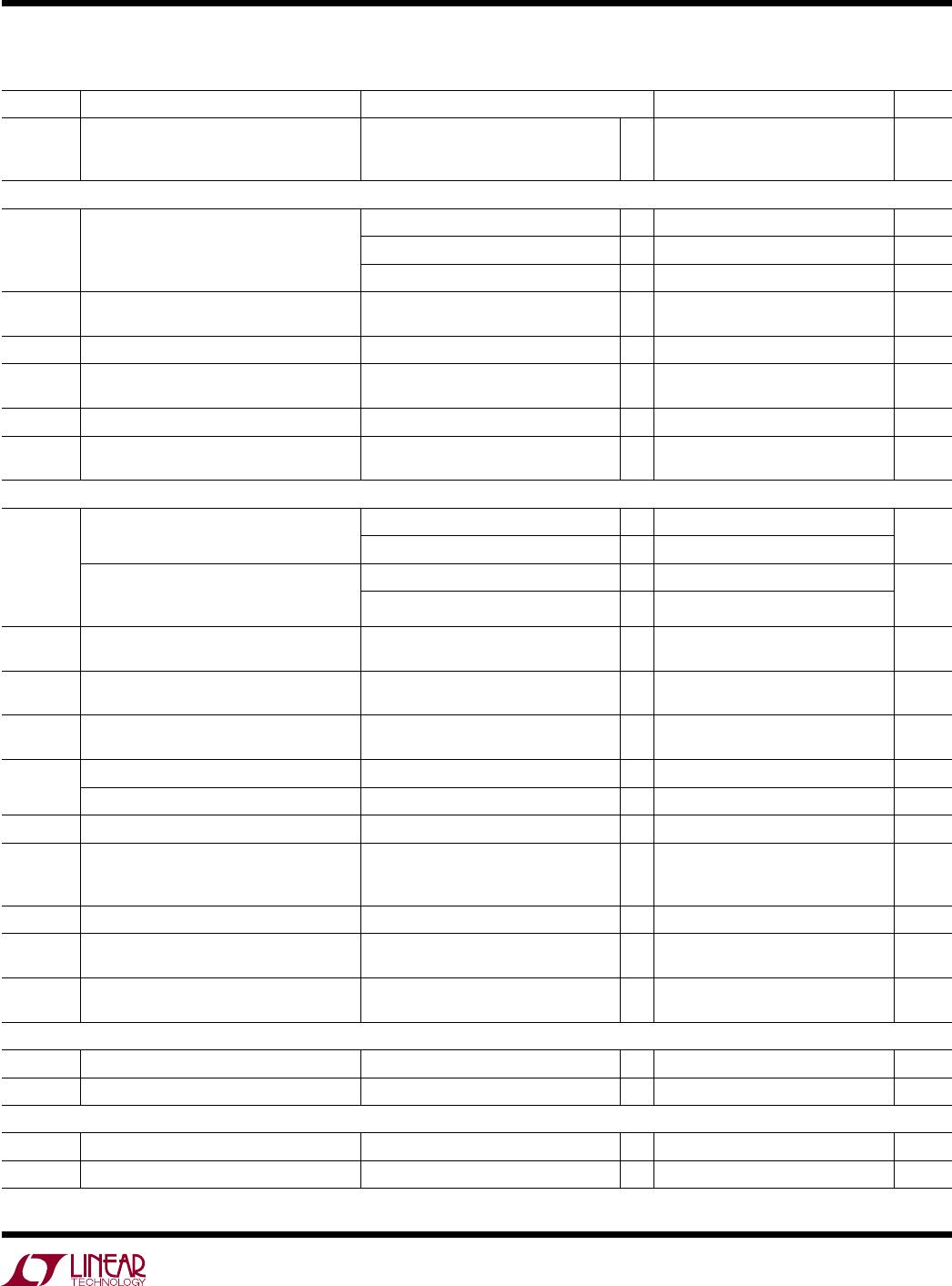# DatasheetLTC2862/LTC2863/
LTC2864/LTC2865
5
2862345fc
SYMBOL PARAMETER CONDITIONS MIN TYP MAX UNITS
I
CCTRS
Supply Current with Both Driver and
LTC2864-2, LTC2865 with SLO Low)
CC
= V
L
, RE = 0V
l
3.3 8 mA
Driver
|V
OD
| Differential Driver Output Voltage R = ∞ (Figure 1)
l
1.5 V
CC
V
R = 27Ω (Figure 1)
l
1.5 5 V
R = 50Ω (Figure 1)
l
2 V
CC
V
Δ|V
OD
| Change in Magnitude of Driver Differential
Output Voltage
R = 27Ω or 50Ω (Figure 1)
l
0.2 V
V
OC
Driver Common-Mode Output Voltage R = 27Ω or 50Ω (Figure 1)
l
3 V
Δ|V
OC
| Change in Magnitude of Driver
Common-Mode Output Voltage
R = 27Ω or 50Ω (Figure 1)
l
0.2 V
I
OSD
Maximum Driver Short-Circuit Current –60V ≤ (Y or Z) ≤ 60V (Figure 2)
l
±150 ±250 mA
I
OZD
Driver Three-State (High Impedance)
Output Current on Y and Z
DE = 0V, V
CC
= 0V or 3.3V, V
O
= –25V,
25V
l
±30 µA
I
IN
V
CC
= 0V or 3.3V, V
IN
= 12V (Figure 3)
l
125 µA
µA
V
CC
= 0V or 3.3V, V
IN
= –7V (Figure 3)
l
–100
LTC2865; C-, I-, H-, MP-Grade LTC2862)
V
CC
= 0V or 3.3V, V
IN
= 12V (Figure 3)
l
143 µA
µA
V
CC
= 0V or 3.3V, V
IN
= –7V (Figure 3)
l
–100
R
IN
Receiver Input Resistance 0 ≤ V
CC
≤ 5.5V, V
IN
= –25V or 25V
(Figure 3)
112
V
CM
(A + B)/2
l
–25 25 V
V
TH
Differential Input Signal Threshold
Voltage (A – B)
–25V ≤ V
CM
≤ 25V
l
±200 mV
ΔV
TH
Differential Input Signal Hysteresis V
CM
= 0V 150 mV
Differential Input Failsafe Threshold Voltage –25V ≤ V
CM
≤ 25V
l
–200 –50 0 mV
Differential Input Failsafe Hysteresis V
CM
= 0V 25 mV
V
OH
Receiver Output High Voltage I(RO) = –3mA (Sourcing)
V
L
≥ 2.25V, I(RO) = –3mA (LTC2865)
V
L
< 2.25V, I(RO) = –2mA (LTC2865)
l
l
l
V
CC
–0.4V
V
L
–0.4V
V
L
–0.4V
V
V
OL
Receiver Output Low Voltage I(RO) = 3mA (Sinking)
l
0.4 V
I
OZR
Output Current on RO
RE = High, RO = 0V or V
CC
RO = 0V or V
L
(LTC2865)
l
±5 µA
I
OSR
Receiver Short-Circuit Current RE = Low, RO = 0V or V
CC
RO = 0V or V
L
(LTC2865)
l
±20 mA
Logic (LTC2862, LTC2863, LTC2864)
V
TH
Input Threshold Voltage (DE, DI, RE) 3.0 ≤ V
CC
≤ 5.5V
l
0.33V
CC
0.67 • V
CC
V
I
INL
Logic Input Current (DE, DI, RE) 0 ≤ V
IN
≤ V
CC
l
0 ±5 µA
Logic (LTC2865)
V
TH
Input Threshold Voltage (DE, DI, RE, SLO) 1.65V ≤ V
L
≤ 5.5V
l
0.33V
L
0.67V
L
V
I
INL
Logic Input Current (DE, DI, RE, SLO) 0 ≤ V
IN
≤ V
L
l
0 ±5 µA
elecTrical characTerisTics
The l denotes the specifications which apply over the full operating
temperature range, otherwise specifications are at T
A
= 25°C. V
CC
= V
L
= 3.3V unless otherwise noted. (Note 2)